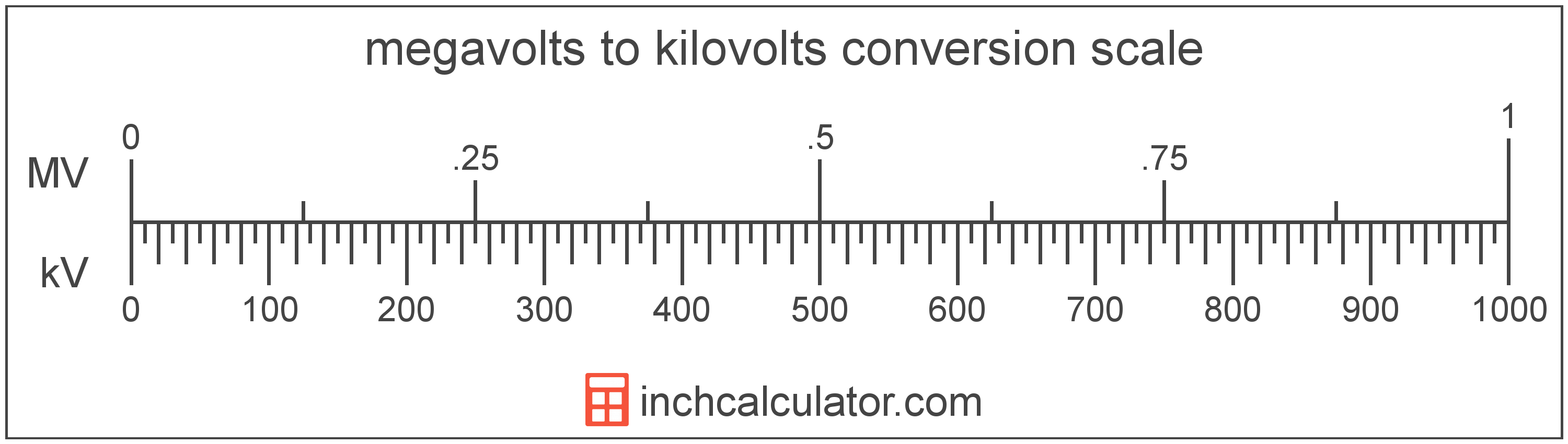# Megavolts to Kilovolts Converter

Enter the voltage in megavolts below to get the value converted to kilovolts.

Results in Kilovolts:1 MV = 1,000 kV

Do you want to convert kilovolts to megavolts?

## How to Convert Megavolts to Kilovolts

To convert a measurement in megavolts to a measurement in kilovolts, multiply the voltage by the following conversion ratio: 1,000 kilovolts/megavolt.

Since one megavolt is equal to 1,000 kilovolts, you can use this simple formula to convert:

kilovolts = megavolts × 1,000

The voltage in kilovolts is equal to the voltage in megavolts multiplied by 1,000.

For example, here's how to convert 5 megavolts to kilovolts using the formula above.
kilovolts = (5 MV × 1,000) = 5,000 kV### How Many Kilovolts Are in a Megavolt?

There are 1,000 kilovolts in a megavolt, which is why we use this value in the formula above.

1 MV = 1,000 kV

## What Is a Megavolt?

One megavolt is equal to 1,000,000 volts, which are the potential difference that would move one ampere of current against one ohm of resistance.

The megavolt is a multiple of the volt, which is the SI derived unit for voltage. In the metric system, "mega" is the prefix for millions, or 106. Megavolts can be abbreviated as MV; for example, 1 megavolt can be written as 1 MV.

## What Is a Kilovolt?

One kilovolt is equal to 1,000 volts, which are the potential difference that would move one ampere of current against one ohm of resistance.

The kilovolt is a multiple of the volt, which is the SI derived unit for voltage. In the metric system, "kilo" is the prefix for thousands, or 103. Kilovolts can be abbreviated as kV; for example, 1 kilovolt can be written as 1 kV.

## Megavolt to Kilovolt Conversion Table

Table showing various megavolt measurements converted to kilovolts.
Megavolts Kilovolts
0.001 MV 1 kV
0.002 MV 2 kV
0.003 MV 3 kV
0.004 MV 4 kV
0.005 MV 5 kV
0.006 MV 6 kV
0.007 MV 7 kV
0.008 MV 8 kV
0.009 MV 9 kV
0.01 MV 10 kV
0.02 MV 20 kV
0.03 MV 30 kV
0.04 MV 40 kV
0.05 MV 50 kV
0.06 MV 60 kV
0.07 MV 70 kV
0.08 MV 80 kV
0.09 MV 90 kV
0.1 MV 100 kV
0.2 MV 200 kV
0.3 MV 300 kV
0.4 MV 400 kV
0.5 MV 500 kV
0.6 MV 600 kV
0.7 MV 700 kV
0.8 MV 800 kV
0.9 MV 900 kV
1 MV 1,000 kV